# 自由定制报告¶

Odoo comes with a powerful and easy-to-use reporting framework. The engine allows you to create new reports, such as tax reports, or balance sheets and income statements with specific groupings and layouts.

Activate the developer mode to access the accounting report creation interface.

To create a new report, go to Accounting ‣ Configuration ‣ Management: Accounting Reports. From here, you can either create a root report or a variant.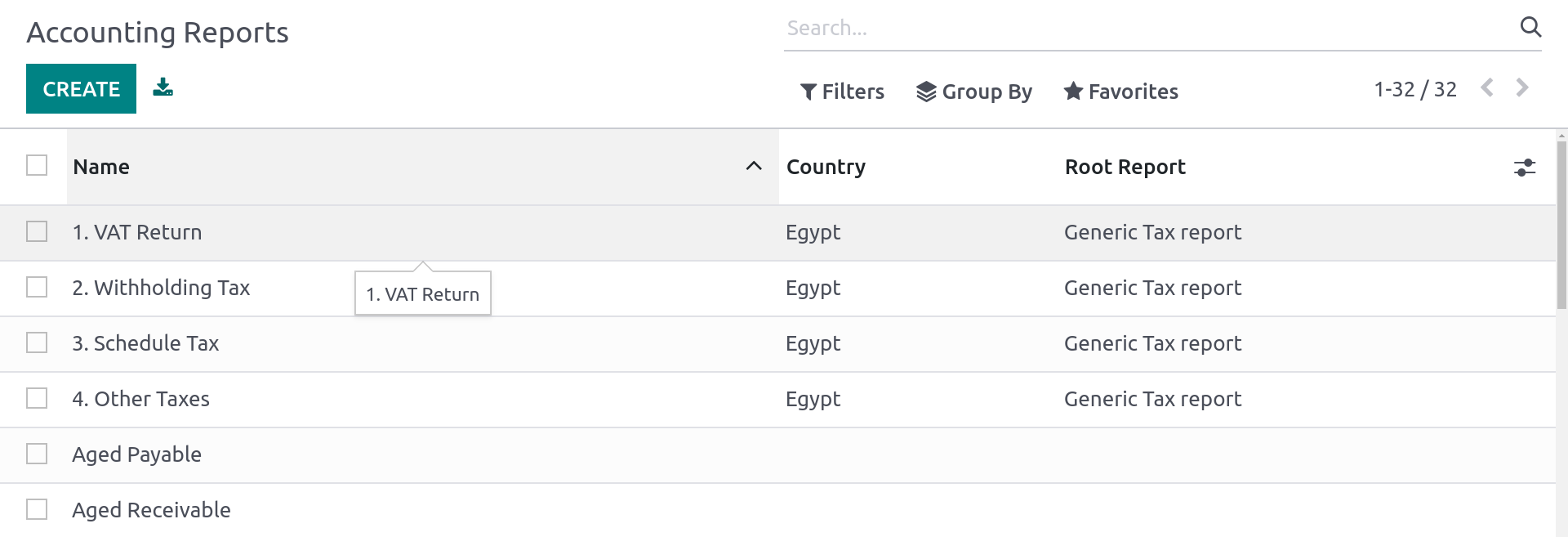## Root reports¶

Root reports can be regarded as generic, neutral accounting reports. They serve as models on which local accounting versions are built. If a report has no root report, it is considered to be a root report itself.

Example

A tax report for Belgium and the US would both use the same generic version as a base and adapt it for their domestic regulations.

When creating a new root report, you need to create a menu item for it. To do so, open the report and then, on that same report, click on Action ‣ Create Menu Item. Refresh the page; the report is now available under Accounting ‣ Reporting.

Cases that require creating a new root report are rare, such as when a country’s tax authorities require a new and specific type of report.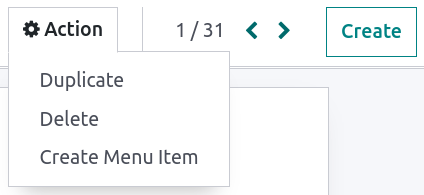## 变体¶

Variants are country-specific versions of root reports and, therefore, always refer to a root report. To create a variant, select a generic (root) report in the Root Report field when creating a new report.

When a root report is opened from one of the accounting app’s main menus, all its variants are displayed in the variant selector in the top right corner of the view.

Example

In the following image, VAT Report (BE) is the variant of the root Generic Tax report.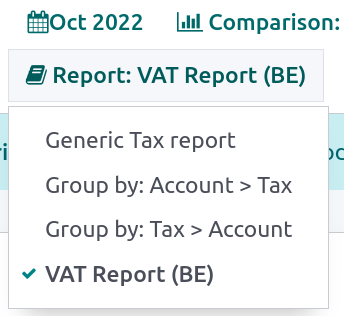## 明细行¶

After having created a report (either root or variant), you need to fill it with lines. You can either create a new one by clicking on Add a line, or modify an existing line by clicking on it. All lines require a Name, and can have an optional additional Code (of your choice) if you wish to use their value in formulas.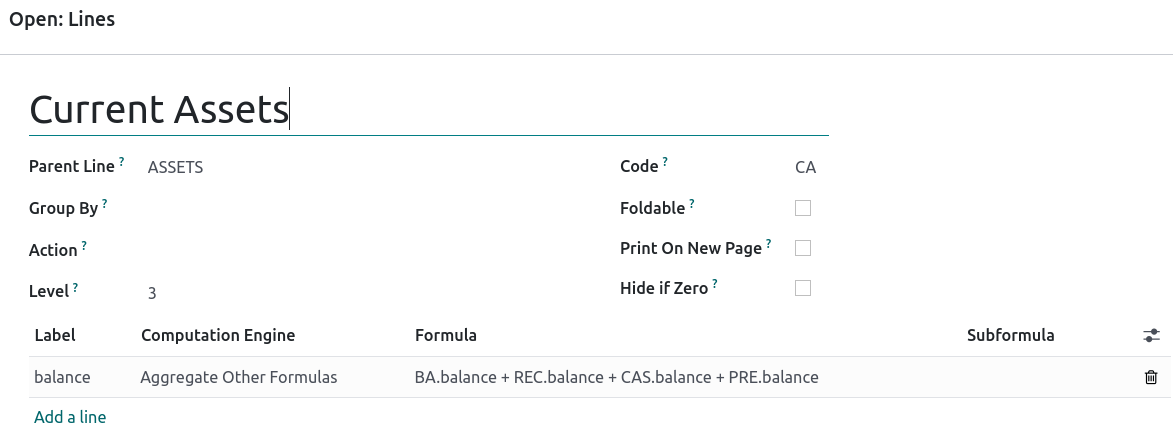## 表达式¶

Each line can contain one or multiple expressions. Expressions can be seen as sub-variables needed by a report line. To create an expression, click on Add a line within a line report.

When creating an expression, you must attribute a label used to refer to that expression. Therefore, it has to be unique among the expressions of each line. Both a Computation Engine and a Formula must also be indicated. The engine defines how your formula(s) and subformula(s) are interpreted. It is possible to mix expressions using different computation engines under the same line if you need to.

Depending on the engine, subformulas may also be required.

### ‘Odoo Domain’ engine¶

With this engine, a formula is interpreted as an Odoo domain targeting `account.move.line` objects.

The subformula allows you to define how the move lines matching the domain are used to compute the value of the expression:

`sum`

The result is the sum of all the balances of the matched move lines.

`sum_if_pos`

The result is the sum of all the balances of the matched move lines if this amount is positive. Otherwise, it is `0`.

`sum_if_neg`

The result is the sum of all the balances of the matched move lines if this amount is negative. Otherwise, it is `0`.

`count_rows`

The result is the number of sub-lines of this expression. If the parent line has a group-by value, this will correspond to the number of distinct grouping keys in the matched move lines. Otherwise, it will be the number of matched move lines.

You can also put a `-` sign at the beginning of the subformula to reverse the sign of the result.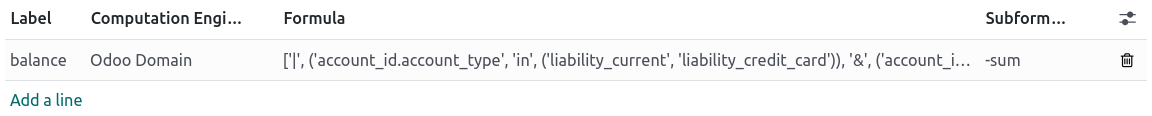### ‘Tax Tags’ engine¶

A formula made for this engine consists of a name used to match tax tags. If such tags do not exist when creating the expression, they will be created.

When evaluating the expression, the expression computation can roughly be expressed as: (amount of the move lines with `+` tag) `-` (amount of the move lines with `-` tag).

Example

If the formula is `tag_name`, the engine matches tax tags `+tag_name` and `-tag_name`, creating them if necessary. To exemplify further: two tags are matched by the formula. If the formula is `A`, it will require (and create, if needed) tags `+A` and `-A`.

### ‘Aggregate Other Formulas’ engine¶

Use this engine when you need to perform arithmetic operations on the amounts obtained for other expressions. Formulas here are composed of references to expressions separated by one of the four basic arithmetic operators (addition `+`, subtraction `-`, division `/`, and multiplication `*`). To refer to an expression, type in its parent line’s code followed by a period `.` and the expression’s label (ex. code.label).

Subformulas can be one of the following:

`if_above(CUR(amount))`

The value of the arithmetic expression will be returned only if it is greater than the provided bound. Otherwise, the result will be `0`.

`if_below(CUR(amount))`

The value of the arithmetic expression will be returned only if it is lower than the provided bound. Otherwise, the result will be `0`.

`if_between(CUR1(amount1), CUR2(amount2))`

The value of the arithmetic expression will be returned only if it is strictly between the provided bounds. Otherwise, it will be brought back to the closest bound.

`if_other_expr_above(LINE_CODE.EXPRESSION_LABEL, CUR(amount))`

The value of the arithmetic expression will be returned only if the value of the expression denoted by the provided line code and expression label is greater than the provided bound. Otherwise, the result will be `0`.

`if_other_expr_below(LINE_CODE.EXPRESSION_LABEL, CUR(amount))`

The value of the arithmetic expression will be returned only if the value of the expression denoted by the provided line code and expression label is lower than the provided bound. Otherwise, the result will be `0`.

`CUR` is the currency code in capital letters, and `amount` is the amount of the bound expressed in that currency.

You can also use the `cross_report` subformula to match an expression found in another report.

### ‘Prefix of Account Codes’ engine¶

This engine is used to match amounts made on accounts using the prefixes of these accounts’ codes as variables in an arithmetic expression.

Example

`21`
Arithmetic expressions can also be a single prefix, such as here.

Example

`21 + 10 - 5`
This formula adds the balances of the move lines made on accounts whose codes start with `21` and `10`, and subtracts the balance of the ones on accounts with the prefix `5`.

It is also possible to ignore a selection of sub-prefixes.

Example

`21 + 10\(101, 102) - 5\(57)`
This formula works the same way as the previous example but ignores the prefixes `101`, `102`, and `57`.

You can apply ‘sub-filtering’ on credits and debits using the `C` and `D` suffixes. In this case, an account will only be considered if its prefix matches, and if the total balance of the move lines made on this account is credit/debit.

Example

Account `210001` has a balance of -42 and account `210002` has a balance of 25. The formula `21D` only matches the account `210002`, and hence returns 25. `210001` is not matched, as its balance is credit.

Prefix exclusions can be mixed with the `C` and `D` suffixes.

Example

`21D + 10\(101, 102)C - 5\(57)`
This formula adds the balances of the move lines made on accounts whose code starts with `21` if it is debit (`D`) and `10` if it is credit (`C`), but ignores prefixes `101`, `102`, and subtracts the balance of the ones on accounts with the prefix `5`, ignoring the prefix `57`.

To match the letter `C` or `D` in a prefix and not use it as a suffix, use an empty exclusion `()`.

Example

`21D\()`
This formula matches accounts whose code starts with `21D`, regardless of their balance sign.

### ‘External Value’ engine¶

The ‘external value’ engine is used to refer to manual and carryover values. Those values are not stored using `account.move.line`, but with `account.report.external.value`. Each of these objects directly points to the expression it impacts, so very little needs to be done about their selection here.

Formulas can be one of the following:

`sum`

If the result must be the sum of all the external values in the period.

`most_recent`

If the result must be the value of the latest external value in the period.

In addition, subformulas can be used in two ways:

`rounding=X`

Replacing `X` with a number instructs to round the amount to X decimals.

`editable`

Indicates this expression can be edited manually, triggering the display of an icon in the report, allowing the user to perform this action.

Manual values are created at the `date_to` currently selected in the report.

Both subformulas can be mixed by separating them with a `;`.

Example

`editable;rounding=2`
is a correct subformula mixing both behaviors.

### ‘Custom Python Function’ engine¶

This engine is a means for developers to introduce custom computation of expressions on a case-by-case basis. The formula is the name of a python function to call, and the subformula is a key to fetch in the dictionary returned by this function. Use it only if you are making a custom module of your own.

## 列¶

Reports can have an indefinite number of columns to display. Each column gets its values from the expressions declared on the lines. The field expression_label of the column gives the label of the expressions whose value is displayed. If a line has no expression in that field, then nothing is displayed for it in this column. If multiple columns are required, you must use different expression labels.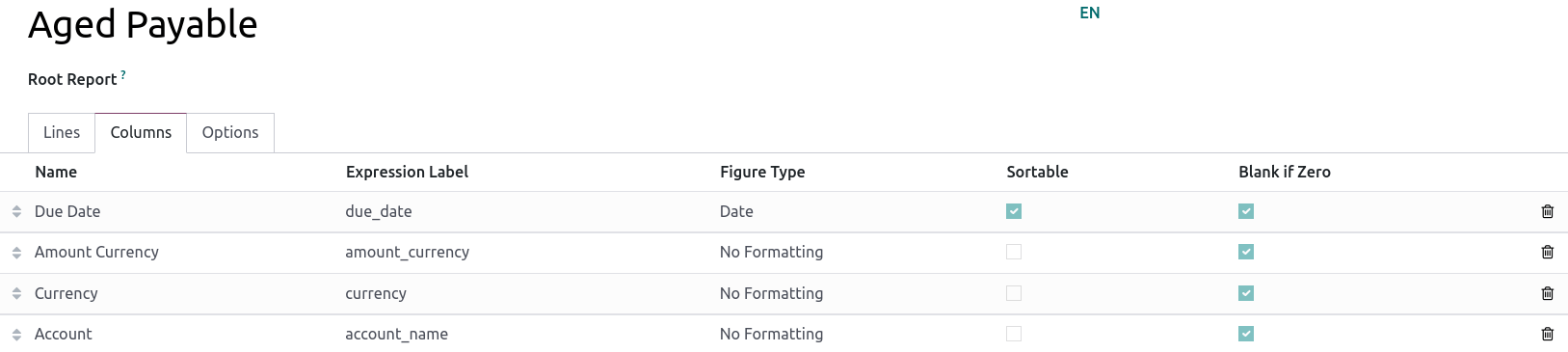When using the period comparison feature found under the Options tab of an accounting report, all columns are repeated in and for each period.